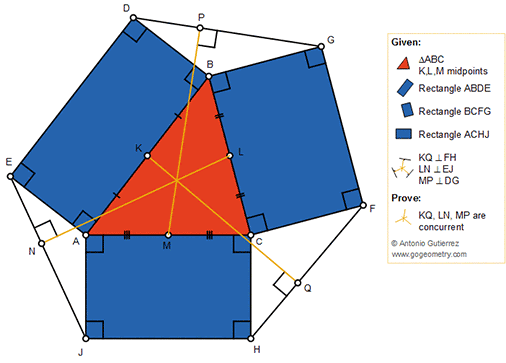## Wednesday, October 24, 2018

### Geometry Problem 1395: Triangle with three rectangles on the sides, Midpoints, Perpendicular lines, Concurrency

Geometry Problem. Post your solution in the comment box below.
Level: Mathematics Education, High School, Honors Geometry, College.

Details: Click on the figure below.#### 1 comment:

1.https://photos.app.goo.gl/3a76E7ztEMNkD1fw6

Let u, v, x, y , w, z are angles of triangles HCF, BDG and AEJ as shown in the sketch
In triangle HCF draw altitude Q’Ct
Note that ∠ (BCt)= u and ∠ (ACt)= v ( angles formed by perpendicular lines)
We also have ∠ (MKQ)= ∠ (BCt)= u ( angles formed by parallel lines)
And ∠ (LKQ)= ∠ (ACt)= v
Similarly we also have x, y,w, z inside triangle KLM as shown
We have sin(u)/sin(v)= CH/CF , sin(y)/sin(x)= BD/BG and sin(w)/sin(z)= AJ/AE
Multiply 3 expressions side by side and note that ABDE, BCFG and ACHJ are the rectangles
We have sin(u)/sin(v) * sin ( y)/sin(x) * sin(w)/sin(z)= 1
So KQ , NL and MP are concurrent per Ceva’s theorem